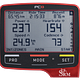# Introduction

Update march 6 2018: Alex Simmons pointed out that CTL and ATL are calculated as exponential moving averages of TSS and not straight averages. I corrected the calculations.

This article will give you the formulas (or ‘algorithms’) for several metrics that are introduced or explained in the book ‘Training and Racing with a Power Meter’ by Hunter and Allen:

• Normalized Power
• Intensity Factor
• Training Stress Score
• Chronic Training Load
• Acute Training Load
• Training Stress Balance
• Variability Index

I wrote this article because I searched for something like this but could not find it. It would have been useful for me and I hope it will be for you.

Normalized Power (NP) is a metric to quantify training intensity with power data and is introduced by Andrew Coggan. The concept of NP is discussed in chapter 7 of the book. It is especially useful in conjuction with the other algorithms below.

Step 1: Calculate the rolling average with a window of 30 seconds: Start at 30 seconds, calculate the average power of the previous 30 seconds and to the for every second after that.

Step 2: Calculate the 4th power of the values from the previous step.

Step 3: Calculate the average of the values from the previous step.

Step 4: Take the fourth root of the average from the previous step. This is your normalized power.

Or in pseudo code:

rolling_average = 30 second rolling average

rolling_avg_powered = rolling_average^4

avg_powered_values = average of rolling_avg_powered

NP = avg_powered_values^0.25

The unit of NP is Watt.

Intensity Factor (IF) is a the ratio of the Normalized Power (NP, see above) with your Functional Threshold Power (FTP). The concept of IF is introduced by Andrew Coggan and is discussed in chapter 7 of the book.

IF = NP / FTP

Where:

• IF = Intensity Factor
• NP = Normalized Power
• FTP = Functional Threshold Power

IF has no unit, it is dimensionless.

Training Stress Score is a metric to quantify training load. It is dimensionless and adapts to changing fitness levels (i.e. your FTP). The concept of TSS is introduced by Andrew Coggan and is discussed in chapter 7 of the book.

TSS = ((t * NP * IF) / (FTP * 3600)) * 100

Or, if we would be more efficient than the book:

TSS = (t * NP * IF) / (FTP * 36)

Where:

• TSS = Training Stress Score
• t = duration of workout in seconds
• NP = Normalized Power
• IF = Intensity Factor
• FTP = Functional Threshold Power

TSS has no unit, it is dimensionless.

Chronic Training Load (CTL) is calculated as an exponentially weighted moving average of daily TSS with a default time constant of 42 days. The concept of CTL is introduced by Andrew Coggan and is discussed in chapter 8 of the book. It is really only useful for calculating TSB, see below.

CTL = Average TSS of last 42 days

CTL has no unit, it is dimensionless.

Acute Training Load (ATL) is calculated as an exponentially weighted moving average of daily TSS with a default time constant of 7 days. The concept of ATL is introduced by Andrew Coggan and is discussed in chapter 8 of the book. It is really only useful for calculating TSB, see below.

ATL = Average TSS of last 7 days

ATL has no unit, it is dimensionless.

Training Stress Balance (TSB) is defined as the difference between CTL and ATL. The concept of TSB is introduced by Andrew Coggan and is discussed in chapter 8 of the book.

TSB = CTL – ATL

TSB has no unit, it is dimensionless.

The Variability Index (VI) can be used as a metric for how smoothly paced your workout or race was and is defined as the ratio between NP and average power of a workout. The concept of VI is not introduced by Andrew Coggan but Charles Howe and is discussed in chapter 8 of the book.

VI = NP / P_avg

Where:

• VI = Variability Index
• NP = Normalized Power
• P_avg = Average power

VI has no unit, it is dimensionless.

## Critical Powers

Using science for critical analysis and innovation in…

## Critical Powers

Using science for critical analysis and innovation in sports.

Written by

## Aart Goossens

Freelance sports data engineer. https://gssns.io## Critical Powers

Using science for critical analysis and innovation in sports.

Medium is an open platform where 170 million readers come to find insightful and dynamic thinking. Here, expert and undiscovered voices alike dive into the heart of any topic and bring new ideas to the surface. Learn more

Follow the writers, publications, and topics that matter to you, and you’ll see them on your homepage and in your inbox. Explore

If you have a story to tell, knowledge to share, or a perspective to offer — welcome home. It’s easy and free to post your thinking on any topic. Write on Medium